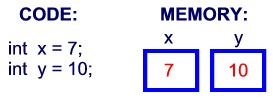# Java long type error: error: integer number too large

Java Basic data Types (let’s start with Java basic data types)
A variable is a memory request to store a value. That is, when creating variables, you need to request space in memory.
The memory management system allocates storage space for variables based on their type, which can only be used to store data of that type.Therefore, by defining different types of variables, you can store integers, decimals, or characters in memory.
The two big data types in Java:
Built-in data types refer to data types

Built-in data type
The Java language provides eight basic types. There are six numeric types (four integers, two floats), one character type, and one Boolean type.
Byte:
A byte data type is an 8-bit, signed integer represented by a binary complement; The minimum is minus 128 times minus 2 to the seventh; The maximum is 127 (2^7-1); The default value is 0; Byte is used in large arrays to save space, replacing integers because byte variables take up only a quarter of the space of an int. Example: Byte a = 100, byte B = -50.
Short:
The minimum value of a 16-bit, signed integer represented by binary complement is -32768 (-2^15). The maximum is 32767 (2^15-1); Short data types can also save as much space as a byte. A short variable is one half of the space occupied by an int variable. The default value is 0; Example: Short s = 1000, short r = -20000.
Int:
The int data type is a 32-bit, signed integer represented as a binary complement; The minimum is -2,147,483,648 (-2^31); The maximum value is 2,147,483,647 (2^ 31-1); Generally, integer variables default to int; The default value is 0; Example: int a = 100000, int B = -200000.
Long:
The long data type is a 64-bit, signed integer represented as a binary complement; The minimum value is – 9223372036854775808 (2 ^ 63); Maximum is 9223372036854775807 (2 ^ 63-1); This type is mainly used on systems that require larger integers; The default value is 0L; Example: long A = 100000L, long B = -200000l.
“L” is not case-sensitive in theory, but if written as “L”, it is easy to confuse with the number “1” and cannot be easily distinguished. So it’s better to capitalize.
Float:
The float data type is a single-precision, 32-bit, IEEE 754 compliant float; Floats save memory when storing large floating point arrays. The default value is 0.0f; Floating-point Numbers cannot be used to represent exact values, such as currency; Example: Float F1 = 234.5F.
Double:
A double data type is a double-precision, 64-bit, IEEE 754 compliant floating point; The default type of floating-point Numbers is double; Double also does not represent exact values, such as currency; The default value is 0.0d; Example: Double D1 = 123.4.
Boolean:
Boolean data types represent bits of information; There are only two values: true and false; This type is used only as a flag to record true/false cases; The default value is false; Example: Boolean One = true.
Char:
Char is a single 16-bit Unicode character; The minimum value is \u0000 (i.e., 0); The maximum value is \ UFFFF (i.e. 65,535); Char data type can store any character; Char letter = ‘A’;

* * * * * * * * * * * * * * * * * * * * * * * * * * * * * * * * * * * * * * * * * * * * * * * * * * * * * * * * * * * * * * * * * * * * * * * * * * * * * * * * * * * * * * * * * * * * * * * * * * * * * * * * * * * * * * * * * * * * * * * * * * * * * * * * * * * * * * * * * * * * * * * * * * * * * * * * * * * * * * * * * * * * * * * * * * * * * * * * * * * * * * * * * * * * * * * * * * * * * * * * * *

Defined in Java:
Long len = 12345678901211;
Result error:
Error: Integer Number too Large: 12345678901211
Correct writing: long len = 12345678901211L;
Reason: The L is treated as long; If you do not add it and treat it as an int, an error will be reported.